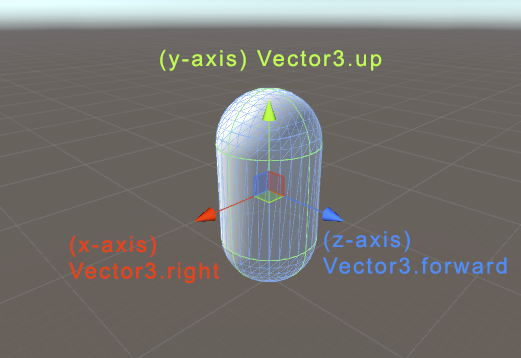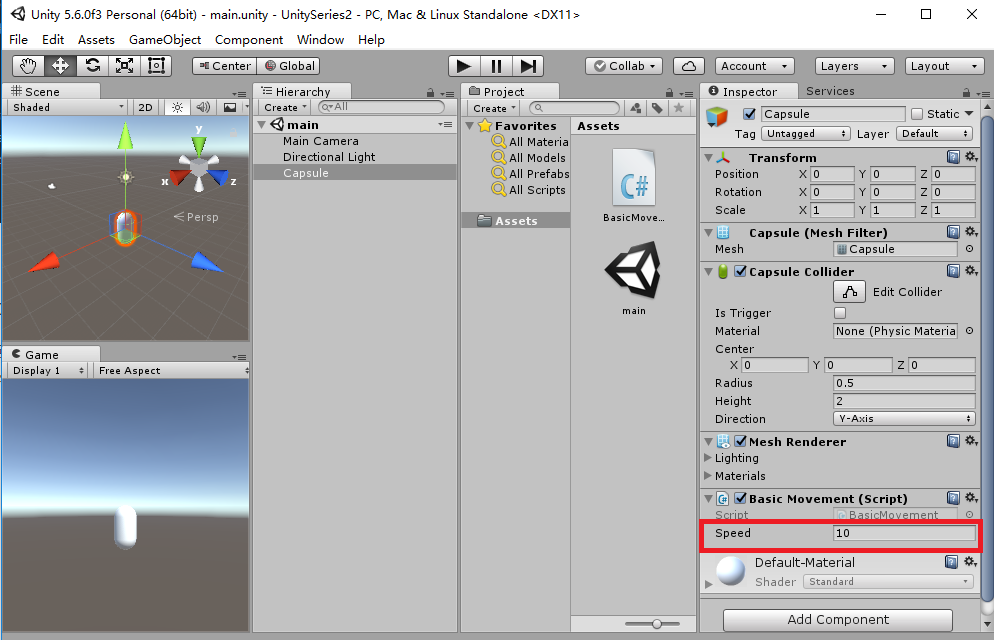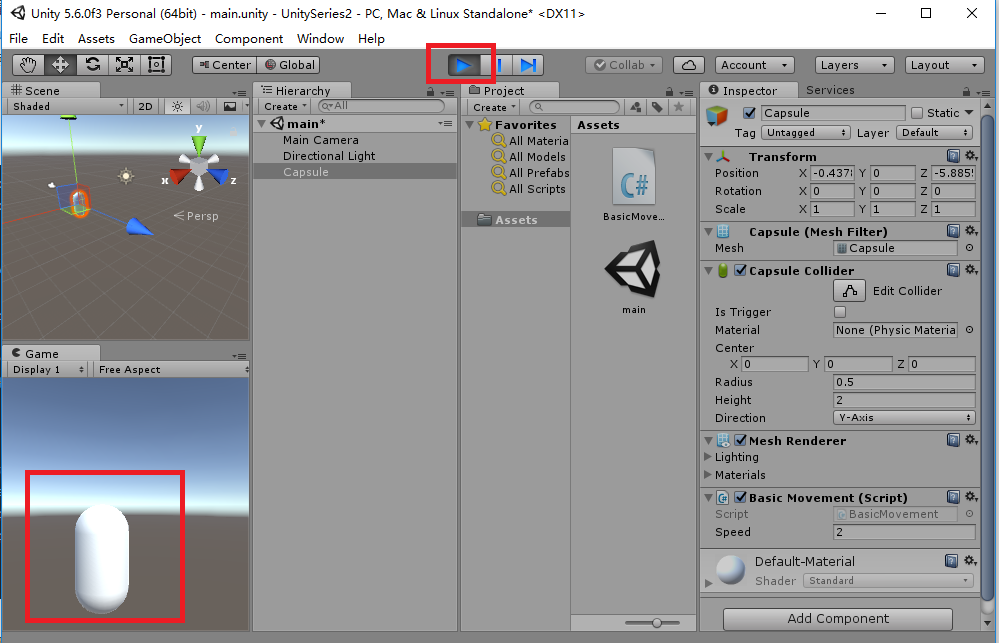# 游戏制作之路（5）玩家控制角色移动// x = 0,y = 1, z = 0
Vector3.up;         //  表示世界坐标系中 Y 轴正方向上的单位向量
// x = 0,y = -1, z = 0
Vector3.down;       //  表示世界坐标系中 Y 轴负方向上的单位向量
// x = -1,y = 0, z = 0
Vector3.left;       //  表示世界坐标系中 X 轴负方向上的单位向量
// x = 1,y = 0, z = 0
Vector3.right;      //  表示世界坐标系中 X 轴正方向上的单位向量
// x = 0,y = 0, z = 1
Vector3.forward;    //  表示世界坐标系中 Z 轴正方向上的单位向量
// x = 0,y = 0, z = -1
Vector3.back;       //  表示世界坐标系中 Z 轴负方向上的单位向量

Vector3.zero;       //  表示原点

using System.Collections;
using System.Collections.Generic;
using UnityEngine;

public class BasicMovement : MonoBehaviour {

public float speed = 10.0f;

// Use this for initialization
void Start () {

}

// Update is called once per frame
void Update () {
transform.position += Vector3.right * Time.deltaTime;
}
}


public float speed = 10.0f;Vector3 input = new Vector3(Input.GetAxisRaw("Horizontal"), 0, Input.GetAxisRaw("Vertical"));

using System.Collections;
using System.Collections.Generic;
using UnityEngine;

public class BasicMovement : MonoBehaviour {

public float speed = 10.0f;

// Use this for initialization
void Start () {

}

// Update is called once per frame
void Update () {
Vector3 input = new Vector3(Input.GetAxisRaw("Horizontal"), 0, Input.GetAxisRaw("Vertical"));
transform.position += input * speed * Time.deltaTime;
}
}### Visual Studio 2015开发C++程序的基本使用 http://edu.csdn.net/course/detail/2570 在VC2015里使用protobuf协议http://edu.csdn.net/course/detail/2582 在VC2015里学会使用MySQL数据库http://edu.csdn.net/course/detail/267208-08
11-19
08-211034
11-168397
05-09
05-232162
07-30
05-05
03-107340
05-26
11-134691
05-085513
09-092401
09-141万+
©️2020 CSDN 皮肤主题: Age of Ai 设计师:meimeiellie点击重新获取扫码支付1.余额是钱包充值的虚拟货币，按照1:1的比例进行支付金额的抵扣。
2.余额无法直接购买下载，可以购买VIP、C币套餐、付费专栏及课程。余额充值